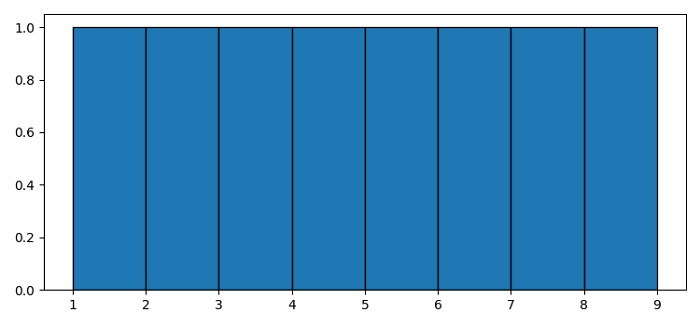# Histogram for discrete values with Matplotlib

To plot a histogram for discrete values with matplotlib, we can use hist() method.

## Steps

• Set the figure size and adjust the padding between and around the subplots.

• Make a list of discrete values.

• Use hist() method to plot data with bins=length of data and edgecolor=black.

• To display the figure, use show() method.

## Example

from matplotlib import pyplot as plt

plt.rcParams["figure.figsize"] = [7.50, 3.50]
plt.rcParams["figure.autolayout"] = True

data = [1, 4, 2, 3, 5, 9, 6, 7]
plt.hist(data, bins=len(data), edgecolor='black')
plt.show()

## Output